﻿ Sensor Circuit Simulation and Power Density Calculation_

China sensor manufacturers

# Sensor Circuit Simulation and Power Density Calculation

Simulation of sensor circuit power consumption and power density calculation results
In this design, the measurement error of the predetermined measurement range from 50 °C to 100 °C is less than -2.8 to 3.8 °C. The adjusted TDC power consumption and the circuit power simulation results shown in the reference are shown in Table 1.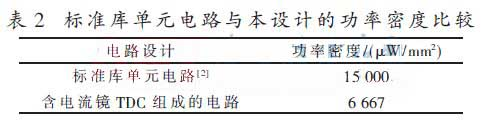It can be seen from Table 1 that the power consumption of the TDC with current mirror is significantly lower than that of the reference. This is mainly because the oscillator's dynamic power calculation formula p=αVdd2fC indicates that the power consumption is positively correlated with the frequency, and the power consumption can be significantly reduced by lowering the frequency.

Directly related to self-heating is power density, which is the power consumption per unit area. The simulated power consumption and circuit area of the circuit can be used to calculate the power density of the circuit based on these results. As shown in Table 2, it can be seen from the calculation that the power density of the TDC in this design is only about 45% of the reference design . This result indicates that the structure of the design will effectively reduce the interference of self-heating on the measurement results.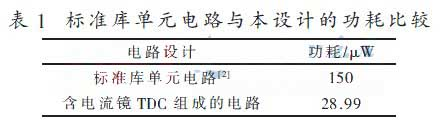Sensor circuit main parameter simulation results
According to the quantitative relationship between the frequency and temperature of the ring oscillator discussed in 1.1, the frequency and temperature are linear, and the simulation results are shown in Fig. 5. As can be seen from the figure, for different process corners, the relationship between frequency and temperature is linear and meets the design requirements.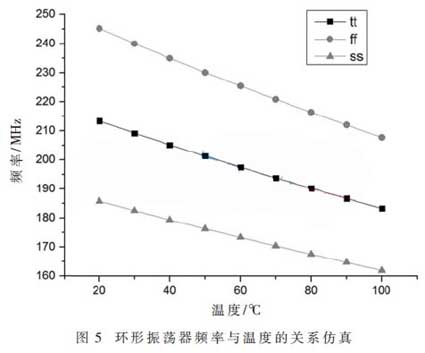The temperature-dependent time variable pulse_width obtained by the sensing portion, the result of the temperature measurement obtained after being quantized by TDC and calibrated by two points, is shown in FIG 6. It can be seen that the measurement error of the sensor can meet the measurement requirements in the temperature range of 50 °C to 100 °C.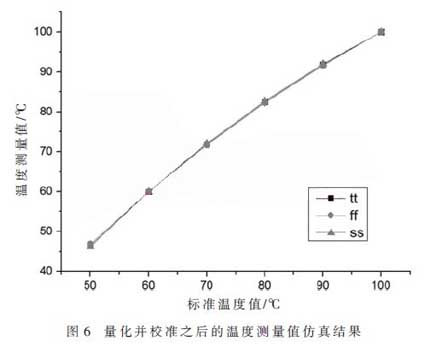The measured error can be obtained by comparing the measured temperature with the standard temperature value, as shown in FIG. It can be seen from Fig. 7 that the temperature deviation is small in the range of 50 °C to 100 °C, and the highest error is only -2.8 °C. It satisfies the design requirement that the error is small within the predetermined measurement range.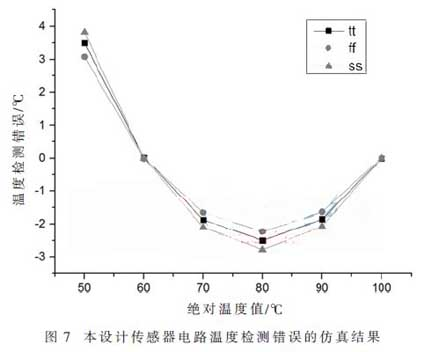3 Conclusion
In this paper, the effect of temperature on the performance of the time domain temperature sensor circuit is improved accordingly. There are two main circuit improvements. The first is to amplify the temperature coefficient by changing the transistor size of the sensing part oscillator, so that the design can meet the accuracy requirements of the application. Secondly, by improving the structure of the TDC oscillation loop and adding a current mirror with current compensation, the temperature drift of the TDC is close to 0 in the controllable measurement range. At the same time, the oscillation frequency of the TDC is reduced, thus the power consumption and self-heating of the circuit are reduced. Finally, the measurement error is reduced to an acceptable range over the application temperature range. Through simulation comparison, the self-heating of this design is reduced by more than half compared with the reference . It can be seen that the delay single with temperature compensation effect to reduce the measurement error will get more attention in future sensors by virtue of its advantages.

﻿
﻿